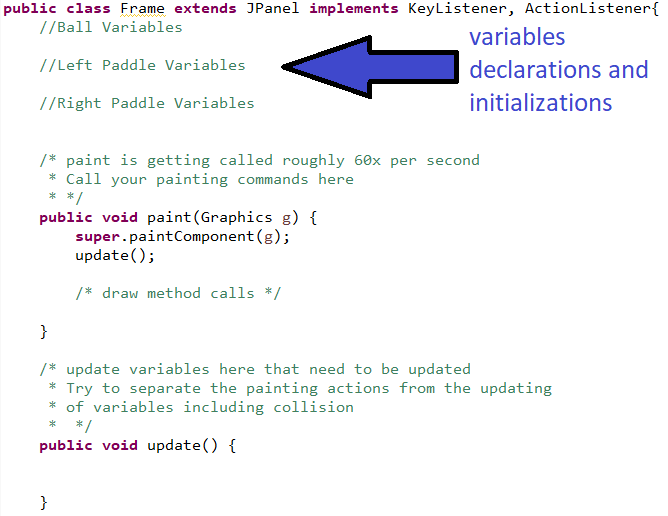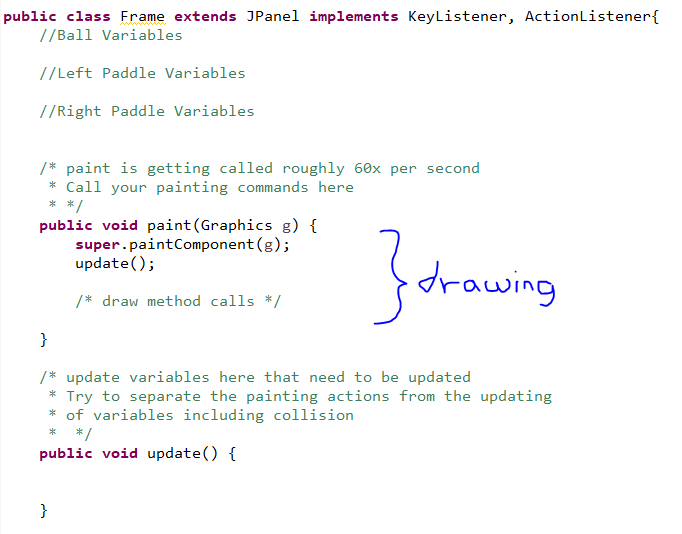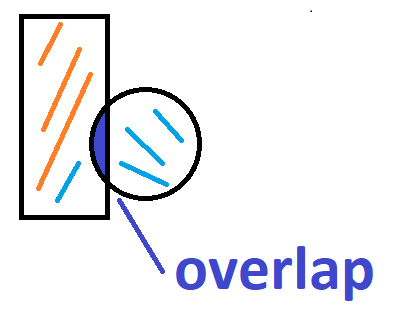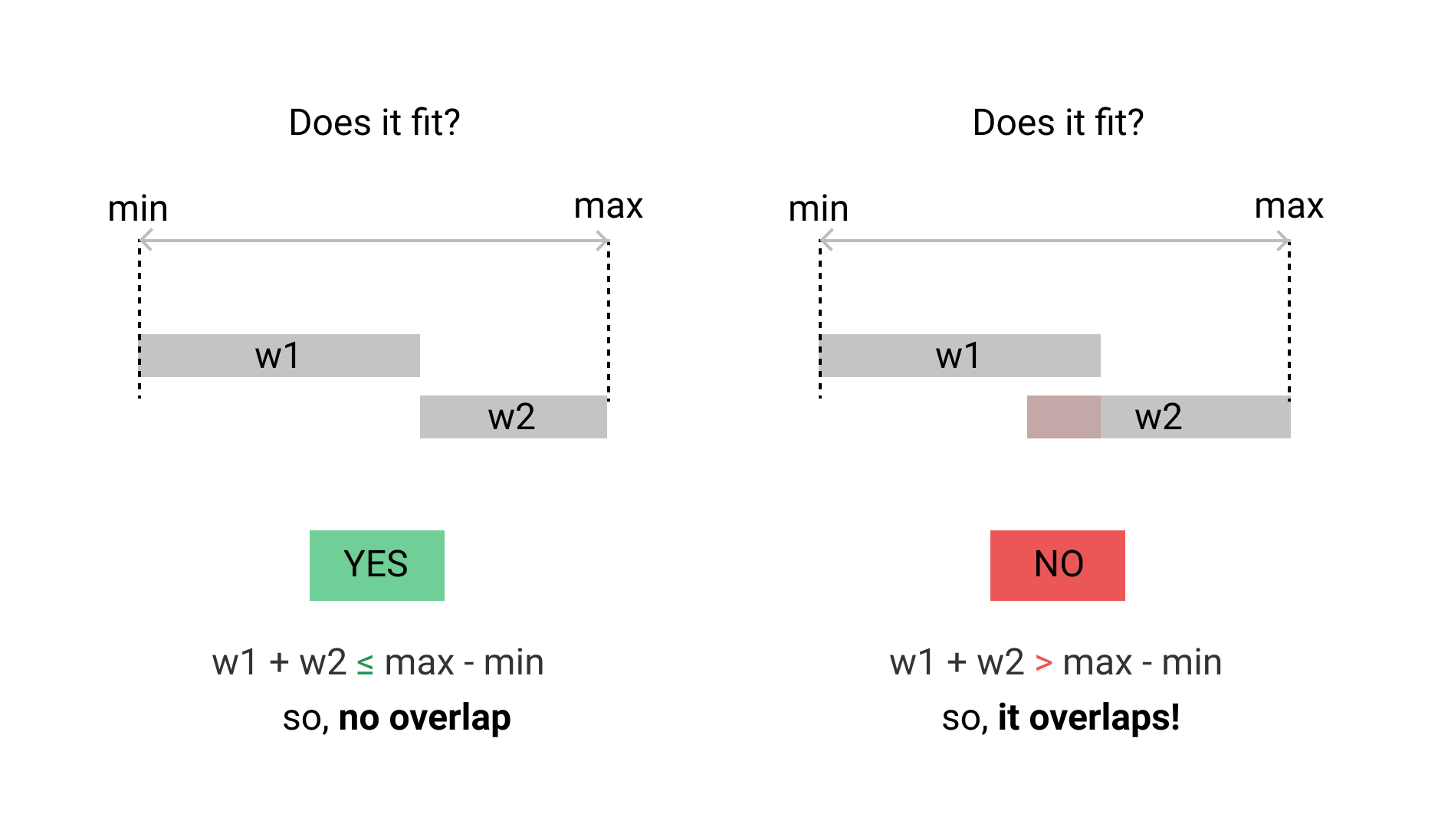### Pong

#### Content Needed to be Successful

Units 1-3
• Variables and Datatypes
• Expressions and Assignment Statements
• Calling Methods with and w/o parameters
• Tracing Methods
• Strings
• Using Math Class
• Boolean Expressions
• If Statements and Control Flow
• Compound Boolean Expressions

#### Pong Game Preview

• Import the Github Project into eclipse

#### Setup

The starter project will integrate easily with eclipse, however, it is a very simplified project. Since it is a one-file project, you can use any IDE without issues! Create any working project in your IDE. In a Frame.java file, you can easily copy-paste the code from GitHub. For anyone using eclipse, clone and the repository and import the project. This project setup has been simplified to targetting 3 areas of the runner file, Frame.java. The three areas will help organize your code.

• Instance Variables: To declare variables needed for the ball, paddles, score, etc.
• paint Method: public void paint() handles all the drawing for the program.
• update Method: public void update() handles updating any variables for the progdrma.

#### Instance Variables

Take a look at Frame.java. Towards the top of the class will be the declaration and instantiation of variables needed for this project. In this simplified setup, the variables at the top as shown below. Drawing will be done in the paint method and updating the variables will be done in the update method. Identify these key areas in the Frame.java file. Do not worry about the rest of the files which deal with running the JFrame. You may learn more about building JFrame-based programs later, but it does not come into play in this project.1. Let's add variables pertaining to a ball's position on the 800x600 screen. We can position it roughly in the center and you can alter the values later so that the ball is centered. Add the following lines of code in the designated area as shown in the picture above. Your variables for the ball and paddles will go here so that they are visible and accessible to the draw and paint methods. If you declare variables inside a method, remember that the scope of the variable is only in the lifetime of that method. In other words, declared variables in one method are not visible to other methods.
```int ballX = 400; //remember that the x-y of an oval in java is actually the top-left corner of the rectangle that would surround the oval
int ballY = 300;```

2. Since the ball has to move, we should add in variables for movement since we want to be able to alter the direction of the ball!
```int ballVx = 3; //x-movement
int ballVy = 3; //y-movement```
3. Finally, let's create a variable for the width of our ball. This can potentially help us alter the width later much faster.
`int ballW = 30;`

Determine variables you will need to represent the left paddle in a game of Pong similar to how you defined the variables for the Ball. Remember that picking descriptive variable names can help you with your programming and debugging.

#### paint MethodThe paint method in this setup runs every 16ms or roughly 60x per second. The reason for this is there is a Timer that calls the function repeatedly. This is going to be used as our animation timer since this is required for games like Pong! The dimension of the Frame is 800x600 pixels which should help when you start placing the paddles and balls on the screen.

To remind you, to draw an oval on the screen you would run the line of code below inside of the paint method.
g.fillOval(50, 50, 100, 100);
Recall that the first 2 arguments are the x, y location of the top-left of the oval. The last 2 arguments are the width and height respectively.

The two main drawing methods we will use are fillOval and fillRect.

 `fillRect(int x, int y, int width, int height)`  Fills the specified rectangle.`fillOval(int x, int y, int width, int height)`   Fills an oval bounded by the specified rectangle with the current color.

To do:
Practice using methods similar to the highlighted line of code shown above to draw a Pong ball in the center.
Practice using methods similar to the highlighted line of code shown above to draw a Pong paddle on the left side of the screen.

Now, instead of hardcoding the values of an oval, you should use the variables you created and initialized for the pong ball as shown below.

`g.fillOval(ballX, ballY, ballW, ballW); `

Determine the draw command needed for the left paddle! Remember to use the "rectangle" rather than the "oval" method!

#### update Method

The update method is where we will separate the part of the code that updates the variables. For example, updating the position of the ball based on the velocity variables will occur in this update method.

To try:
In the update method, try adding the following to increment the x position of the ball.

`ballX++;`

Adding the code above should move the ball to the right over time. Why does this happen? Recall that there is an "animation" timer. The timer will invoke the paint which then invokes the update method. We'll estimate this to happy roughly every 16ms. Over time, this means that x is updated 60x per second so we should observe it increase. Since the variable ballX is being used in the drawing method for the ball, we will see a ball moving to the right over time. After trying the code above, maybe see if you can make the ball go diagonal or in some direction in the y-axis.

Remember that we declared velocity variables for the x and y direction. We should actually use these variables! Why not just hardcode how the ball is moving similar to the discovery activity above? Well, there is not an easy way to change the direction of the ball dynamically if we hardcode how the ball position is moving. If all you had was ballX++; in update, how could you make the ball go to the left later on command? There's not a way without adding the variables for variability!

The ball's velocity in the x direction should affect the ball's x position. The same thing can be said about the y. Add the code below to the update method in placed of what you tried before getting to this point.

```ballX = ballX + ballVx; //velocity x affects x position
ballY = ballY + ballVy; //velocity y affects y position```

Adding the two lines of code above should now have the ball update it's position according to the initial values of ballVx and ballVy.

#### Wall Borders

Let's attempt to change the direction of the ball! If you add the if-statement below, the ball's velocity in the x and y will reverse once the ball reaches towards the positions shown.

```if(ballY > 600){ ballVy *= -1; }
if(ballX > 800){ ballVx *= -1; }```

If you add the code above in the update method, the ball's direction should change at the given x and y positions. You might notice, however, that the ball leaves the frame before it changes direction. Why is this?

The ball's x and y position is actually that of a rectangle that would surround the ball. Specifically, it is the top-left position of said rectangle. In other words, it's not until the left side of the ball leaves the right side of the screen that the condition in the if-statement is met. How can we detect the wall sooner? Well, we want to add an offset to the ball's x position. Since x is the left-side of the ball, adding the width of the ball relative to the current value of x would then always refer to the right side of the ball! The updated if-statement should roughly resemble the lines below unless the width of your ball is slightly different or you're not using the variable we created together.

```if(ballY+ballW > 600){ ballVy *= -1; }
if(ballX+ballW > 600){ ballVx *= -1; }
```

To do:

See if you can debug your code and make the ball bounce when it hits any of the sides of the frame. We will make changes to this logic later as we fine-tune the gameplay to mimic the original game.

#### KeyListener

If you look through the code, you will find several methods dealing with KeyBoard events: keyPressed, keyReleased, and keyTyped. I'll leave it to you to explore the differences in these "events". These methods are attached to events on the JFrame, which is the application window you see when you run the project.

Let's focus on keyPressed for now. When the application window is in focus, it's actively "listening" for key presses. Every key on the keyboard is coded and that code is printed to the console since the first line in the method is a print statement as shown.
`public void keyPressed(KeyEvent key){   System.out.println("Keycode: "+key.getKeyCode());}`

What if you wanted something to happen on the screen when you press the 's' key? For example, perhaps the left paddle moves down?

`public void keyPressed(KeyEvent key){   System.out.println("Keycode: "+key.getKeyCode());   if(key.getKeyCode() == 83){      paddleLeftY += 10;   }}`

In the code above, if I have a variable called paddleLeftY and this variable was used to draw a rectangle on the screen then updating its value to increase would cause the drawing to move down each time I pressed the 's' key which has a keyCode of 83.

What if you want to code other keys, but you don't know what the keyCode is? One easy way (not the only way) of finding out is simply clicking the key you're interested in while running the program and seeing the output to the console. Remember there's a print statement in the method that prints the keyCode when you press a key!

Your turn: How can you add additional if-statements in the keyPressed method to control paddles and have them behave as one might expect? Determine your control scheme for the game. Will you be using the 'w', 'a', 's', 'd' keys, or the arrow keys? Other keys? Up to you!

#### Collision DetectionRudimentary Collision detection can be written by thinking about how to write a boolean expression that checks if the two areas overlap. But how? Well, it's actually just checking if the range of numbers (in the x and y domain) overlap.

The image below summarizes the exact set of conditions necessary to check if there is overlap among the two ranges. Your job is to identify the w1 and w2 which should represent the x ranges of the ball and paddle. You would do the same for the y ranges. The pseudo-code is below.`if( ball_x_range overlaps with paddle_x_range and ball_y_range overlaps with paddle_y_range){    //collision}`The velocity of the ball should reverse in some fashion so that visually it appears as though the ball bounces off the paddle.

#### Scoring

In pong, a user scores if the ball travels past the paddle opposite of them. For example, if player one is on the left side, then they get a point if the ball passes the right paddle because it means the player controlling the right paddle missed the ball.

You should have score variables that correspond to the scores you're tracking. Increment these score variables when you detect the ball passing the respective paddle.

You can display an int variable to the JFrame with the following example drawing command.
`g.drawString(intVar+"", 50, 50);`

The `intVar` above is concatenated to an empty `String` so that it is converted to a String object since the `drawString` method requires a String. The two numbers are the x and y location of the text.

What should happen once the ball passes a paddle? How does a new round start in this game? Does the ball reset? Will you randomize the direction once you have reset the ball position? Where does the ball go at reset?

#### Other Details

Things to consider:
How can you implement a way to make the game harder over time?
How might you implement a high-score system?